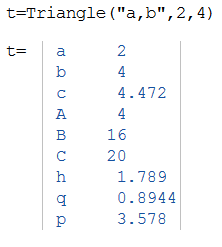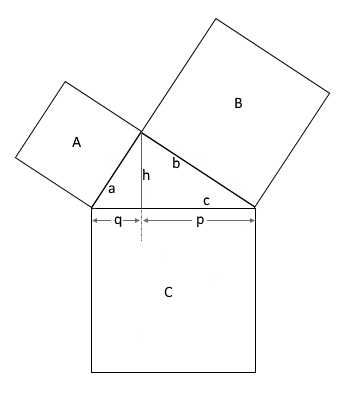# Classes of the RedCrab Calculator

Introduction to the class concept

## Description of the class concept

A class in the RedCrab Calculator is a collection of variables and functions that describe or calculate the properties of a object. These objects may be, for example, geometric figures or other collections of properties such as electrical quantities.

To initialize the object of a class, the class is called like a function. The known properties of the class are passed as parameters. The result is an object that contains the known and unknown properties that can be calculated from the specified arguments.

### Parameters

As a rule, the first parameter is a text that describes the names of the following arguments. The following figure shows the initialization of an object with the properties of a rectangle. The lengths $$a$$ and $$b$$ of the rectangle are given. From this the diagonal $$d$$, the area $$A$$ and the circumference $$P$$ are calculated. The result is an object containing all 5 properties.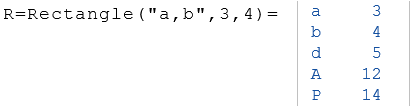The class $$Rectangle$$ always expects 2 arguments. Which properties are passed as an argument does not matter. In the following example the length $$a$$ and the diagonal $$d$$ is given.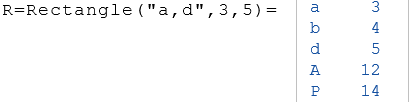If only the value of one properties is of interest, the desired property can be specified in the String Parameter for easy presentation. The string "b-> a, d" in the following example specifies that the length $$b$$ consists of the length $$a$$ and the diagonal $$d$$ should be calculated.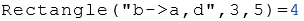The data of an object can be displayed with the equal sign.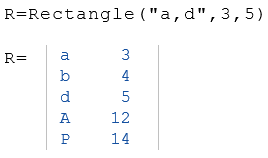The value of a property can be accessed via its symbol, as in the example below. This term can be used anywhere like a variable.Bei einigen Klassen ist eine Eigenschaft als Default voreingestellt. In diesem Fall kann der Wert der voreingestellten Eigenschaft ohne Textstring als Argument übergeben werden. Das Beispiel unten zeigt die Initialisierung der Klasse Circle. Bei Ciscle ist der Radius $$r$$ als Default voreingestellt. For some classes, a property is set as default. In this case, the value of the default property can be passed as an argument without a text string. The example below shows the initialization of the class Circle. The radius $$r$$ of the circle is preset by default.### Example

The following example calculates an object of class Triangle. The symbols of the calculated properties show the drawing next to it.